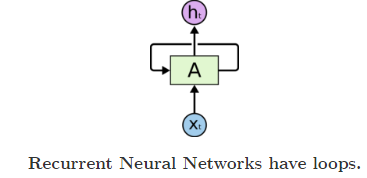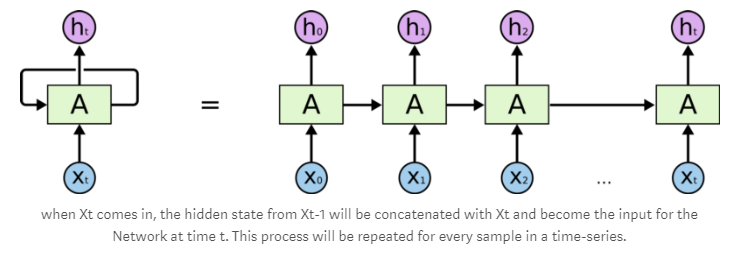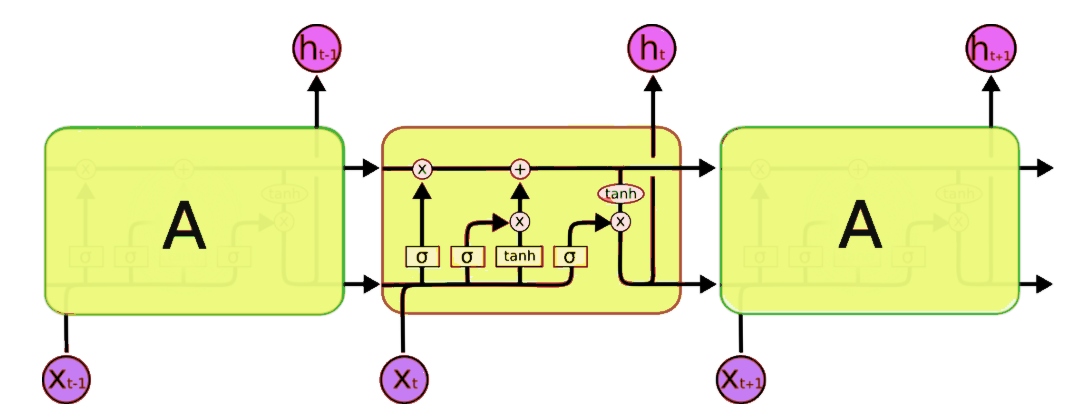×

Search anything:

# Long Short Term Memory (LSTM)

#### Machine Learning (ML) rnn lstmLong short-term memory (LSTM) units are units of a recurrent neural network (RNN). An RNN composed of LSTM units is often called an LSTM network. Hence, we will take a brief look into RNNs.

RNNs are a type of artificial neural network that are able to recognize and predict sequences of data such as text, genomes, handwriting, spoken word, or numerical time series data. They have loops that allow a consistent flow of information and can work on sequences of arbitrary lengths.
To understand RNNs, let’s use a simple perceptron network with one hidden layer. Such a network works well with simple classification problems. As more hidden layers are added, our network will be able to inference more complex sequences in our input data and increase prediction accuracy.

### RNNs structure1. A : Neural Network
2. Xt : Input
3. Ht : Output## 1. What is LSTM?

Long short-term memory (LSTM) units are units of a recurrent neural network (RNN). An RNN composed of LSTM units is often called an LSTM network. A common LSTM unit is composed of a cell, an input gate, an output gate and a forget gate. The cell remembers values over arbitrary time intervals and the three gates regulate the flow of information into and out of the cell.
LSTM networks are well-suited to classifying, processing and making predictions based on time series data, since there can be lags of unknown duration between important events in a time series. LSTMs were developed to deal with the exploding and vanishing gradient problems that can be encountered when training traditional RNNs. Relative insensitivity to gap length is an advantage of LSTM over RNNs, hidden Markov models and other sequence learning methods in numerous applications.

## Need for LSTM

Recurrent Neural Networks work just fine when we are dealing with short-term dependencies. That is when applied to problems like:

`the color of the sky is _______. `

RNNs turn out to be quite effective. This is because this problem has nothing to do with the context of the statement. The RNN need not remember what was said before this, or what was its meaning, all they need to know is that in most cases the sky is blue. Thus the prediction would be:

`the color of the sky is blue. `

However, vanilla RNNs fail to understand the context behind an input. Something that was said long before, cannot be recalled when making predictions in the present. Let’s understand this as an example:

```I spent 20 long years working for the unnder-privileged kids in spain. I then moved to Africa. ...... I can speak fluent _______.```

Here, we can understand that since the author has worked in Spain for 20 years, it is very likely that he may possess a good command over Spanish. But, to make a proper prediction, the RNN needs to remember this context. The relevant information may be separated from the point where it is needed, by a huge load of irrelevant data. This is where a Recurrent Neural Network fails!

The reason behind this is the problem of Vanishing Gradient We know that for a conventional feed-forward neural network, the weight updating that is applied on a particular layer is a multiple of the learning rate, the error term from the previous layer and the input to that layer. Thus, the error term for a particular layer is somewhere a product of all previous layer's errors. When dealing with activation functions like the sigmoid function, the small values of its derivatives (occurring in the error function) gets multiplied multiple times as we move towards the starting layers. As a result of this, the gradient almost vanishes as we move towards the starting layers, and it becomes difficult to train these layers.

A similar case is observed in Recurrent Neural Networks. RNN remembers things for just small durations of time, i.e. if we need the information after a small time it may be reproducible, but once a lot of words are fed in, this information gets lost somewhere. This issue can be resolved by applying a slightly tweaked version of RNNs – the Long Short-Term Memory Networks.

### Architecture of LSTMsThe symbols used here have following meaning:

1. X : Scaling of information

3. σ : Sigmoid layer

4. tanh: tanh layer

5. h(t-1) : Output of last LSTM unit

6. c(t-1) : Memory from last LSTM unit

7. X(t) : Current input

8. c(t) : New updated memory

9. h(t) : Current output

## Area of application

1. Speech recognition
2. Video synthesis
3. Natural language processing
4. Language modeling
5. Machine Translation also known as sequence to sequence learning
6. Image captioning
7. Hand writing generation
8. Image generation using attention models
10. Video to text
• A quick video tutorial on LSTM

### Sample, Timesteps and Features

Name Definetion
Sample This is the len(data_x), or the amount of data points you have.
timesteps This is equivalent to the amount of time steps you run your recurrent neural network. If you want your network to have memory of 60 characters, this number should be 60.
Features this is the amount of features in every time step. If you are processing pictures, this is the amount of pixels.